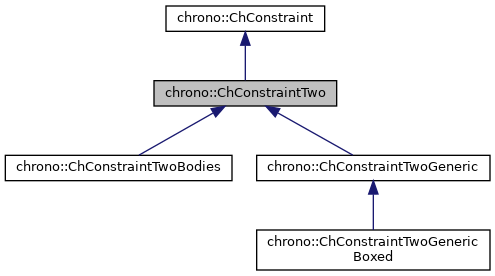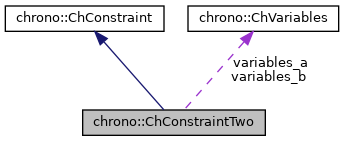chrono::ChConstraintTwo Class Referenceabstract

## Description

This class implements the functionality for a constraint between a COUPLE of TWO objects of type ChVariables(), and defines two constraint matrices, whose column number automatically matches the number of elements in variables vectors.

Before starting the solver one must provide the proper values in constraints (and update them if necessary), i.e. must set at least the c_i and b_i values, and jacobians.

#include <ChConstraintTwo.h>

Inheritance diagram for chrono::ChConstraintTwo:[legend]
Collaboration diagram for chrono::ChConstraintTwo:[legend]

## Public Member Functions

ChConstraintTwo ()
Default constructor.

ChConstraintTwo (const ChConstraintTwo &other)
Copy constructor.

ChConstraintTwooperator= (const ChConstraintTwo &other)
Assignment operator: copy from other object.

virtual ChRowVectorRef Get_Cq_a ()=0
Access jacobian vector.

virtual ChRowVectorRef Get_Cq_b ()=0
Access jacobian vector.

virtual ChVectorRef Get_Eq_a ()=0
Access auxiliary vector (ex: used by iterative solvers).

virtual ChVectorRef Get_Eq_b ()=0
Access auxiliary vector (ex: used by iterative solvers).

ChVariablesGetVariables_a ()
Access the first variable object.

ChVariablesGetVariables_b ()
Access the second variable object.

virtual void SetVariables (ChVariables *mvariables_a, ChVariables *mvariables_b)=0
Set references to the constrained objects, each of ChVariables type, automatically creating/resizing jacobians if needed.

virtual void ArchiveOUT (ChArchiveOut &marchive) override
Method to allow serialization of transient data to archives.

virtual void ArchiveIN (ChArchiveIn &marchive) override
Method to allow de-serialization of transient data from archives.Public Member Functions inherited from chrono::ChConstraint
ChConstraint ()
Default constructor.

ChConstraint (const ChConstraint &other)
Copy constructor.

virtual ChConstraintClone () const =0
"Virtual" copy constructor.

ChConstraintoperator= (const ChConstraint &other)
Assignment operator: copy from other object.

bool operator== (const ChConstraint &other) const
Comparison (compares only flags, not the jacobians etc.)

bool IsValid () const
Tells if the constraint data is currently valid.

void SetValid (bool mon)
Use this function to set the valid state (child class Children classes must use this function depending on the result of their implementations of RestoreReference();.

bool IsDisabled () const
Tells if the constraint is currently turned on or off by the user.

void SetDisabled (bool mon)
User can use this to enable/disable the constraint as desired.

bool IsRedundant () const
Tells if the constraint is redundant or singular.

void SetRedundant (bool mon)
Solvers may use the following to mark a constraint as redundant.

bool IsBroken () const
Tells if the constraint is broken, for excess of pulling/pushing.

void SetBroken (bool mon)
3rd party software can set the 'broken' status via this method (by default, constraints never break);

virtual bool IsUnilateral () const
Tells if the constraint is unilateral (typical complementarity constraint).

virtual bool IsLinear () const
Tells if the constraint is linear (if non linear, returns false).

eChConstraintMode GetMode () const
Gets the mode of the constraint: free / lock / complementary A typical constraint has 'lock = true' by default.

void SetMode (eChConstraintMode mmode)
Sets the mode of the constraint: free / lock / complementary.

bool IsActive () const
Tells if the constraint is currently active, in general, that is tells if it must be included into the system solver or not. More...

void SetActive (bool isactive)
Set the status of the constraint to active.

virtual double Compute_c_i ()
Compute the residual of the constraint using the LINEAR expression. More...

double Get_c_i () const
Return the residual 'c_i' of this constraint. // CURRENTLY NOT USED.

void Set_b_i (const double mb)
Sets the known term b_i in [Cq_i]*q + b_i = 0, where: c_i = [Cq_i]*q + b_i = 0.

double Get_b_i () const
Return the known term b_i in [Cq_i]*q + b_i = 0, where: c_i= [Cq_i]*q + b_i = 0.

void Set_cfm_i (const double mcfm)
Sets the constraint force mixing term (default=0). More...

double Get_cfm_i () const
Returns the constraint force mixing term.

void Set_l_i (double ml_i)
Sets the 'l_i' value (constraint reaction, see 'l' vector)

double Get_l_i () const
Return the 'l_i' value (constraint reaction, see 'l' vector)

virtual void Update_auxiliary ()
This function must update jacobians and auxiliary data such as the 'g_i' product. More...

double Get_g_i () const
Return the 'g_i' product , that is [Cq_i]*[invM_i]*[Cq_i]' (+cfm)

void Set_g_i (double m_g_i)
Usually you should not use the Set_g_i function, because g_i should be automatically computed during the Update_auxiliary() .

virtual double Compute_Cq_q ()=0
This function must computes the product between the row-jacobian of this constraint '[Cq_i]' and the vector of variables, 'q', that is, Cq_q=[Cq_i]*q. More...

virtual void Increment_q (const double deltal)=0
This function must increment the vector of variables 'q' with the quantity [invM]*[Cq_i]'deltal,that is q+=[invM][Cq_i]'*deltal This is used for some iterative solvers. More...

virtual void MultiplyAndAdd (double &result, const ChVectorDynamic< double > &vect) const =0
Computes the product of the corresponding block in the system matrix by 'vect', and add to 'result'. More...

virtual void MultiplyTandAdd (ChVectorDynamic< double > &result, double l)=0
Computes the product of the corresponding transposed block in the system matrix (ie. More...

virtual void Project ()
For iterative solvers: project the value of a possible 'l_i' value of constraint reaction onto admissible orthant/set. More...

virtual double Violation (double mc_i)
Given the residual of the constraint computed as the linear map mc_i = [Cq]*q + b_i + cfm*l_i , returns the violation of the constraint, considering inequalities, etc. More...

virtual void Build_Cq (ChSparseMatrix &storage, int insrow)=0
Puts the jacobian portions into the 'insrow' row of a sparse matrix, where each portion of jacobian is shifted in order to match the offset of the corresponding ChVariable.

virtual void Build_CqT (ChSparseMatrix &storage, int inscol)=0
Same as Build_Cq, but puts the transposed jacobian row as a column.

void SetOffset (int moff)
Set offset in global q vector (set automatically by ChSystemDescriptor)

int GetOffset () const
Get offset in global q vector.

## Protected Attributes

ChVariablesvariables_a
The first constrained object.

ChVariablesvariables_b
The second constrained object.Protected Attributes inherited from chrono::ChConstraint
double c_i
The 'c_i' residual of the constraint (if satisfied, c must be 0)

double l_i
The 'l_i' lagrangian multiplier (reaction)

double b_i
The 'b_i' right term in [Cq_i]*q+b_i=0 , note: c_i= [Cq_i]*q + b_i.

double cfm_i
The constraint force mixing, if needed (usually is zero) to add some numerical 'compliance' in the constraint, that is the equation becomes: c_i= [Cq_i]*q + b_i + cfm*l_i =0; Example, it could be cfm = [k * h^2](^-1) where k is stiffness.

eChConstraintMode mode
mode of the constraint: free / lock / complementar

double g_i
'g_i' product [Cq_i]*[invM_i]*[Cq_i]' (+cfm)

int offset
offset in global "l" state vector (needed by some solvers)

The documentation for this class was generated from the following files:
• /builds/uwsbel/chrono/src/chrono/solver/ChConstraintTwo.h
• /builds/uwsbel/chrono/src/chrono/solver/ChConstraintTwo.cpp